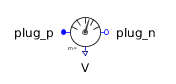Relief Valve with Pressure-Dependent Area - MapleSim Help

Relief Valve with Pressure-Dependent Area

Relief valve with opening area computed from table of pressure vs. areaDescription

The Relief Valve with Pressure-Dependent Area (or Relief Valve Pressure Dependent Area) component models the flow through an orifice where the relationship between the area of the orifice and the pressure difference between port A and port B is supplied in the form of a table. Based on the orifice area, the pressure vs flow rate relationships are derived by the formulation used in the Orifice component.Pressure vs Area For this component, the orifice area must be specified as a function of the pressure difference in the form of a table. The following parameters allows users to specify the necessary data for this calculation. Data Source: This parameter allows users to specify the nature of the source file for the Pressure vs. Area table. The available options are file, attachment, and inline. file: Use this option to specify a source file (an Excel or a CSV file) located on the hard drive. Use the $A\left(\mathrm{dP}\right)$ parameter to browse to and/or select the data file. The data file will not be attached to the MapleSim model. attachment: Use this option to specify a data file (an Excel or a CSV file) that is attached to the MapleSim model. To be used as an attachment, data files must be attached to the MapleSim model and be categorized under Data Sets. The attached files in a MapleSim model can be viewed in the Attachments pane under the Data Sets section. For more information, see Attaching a File to a Model. If the required file is not already attached to the MapleSim model, use the $A\left(\mathrm{dP}\right)$ parameter to attach a new file. Note: If the file option is used, subsequent changes to the source file will be incorporated into MapleSim simulations If the attachment option is used, subsequent changes to the source file will not affect the version attached to the MapleSim model. To ensure updates to the source files are incorporated in the simulation, the file must be reattached. The Data Generation App can be used to generate table data from mathematical functions using Maple code. For more information about MapleSim apps, see Opening MapleSim Apps and Templates. inline: Use this option to directly specify the pressure vs. area relationship in the form of a table. In the $A\left(\mathrm{dP}\right)$ parameter, enter an $m×2$  matrix. The first column must contain values of pressure difference and the second column must contain corresponding values of the area. Note: To change the dimensions of table, right-click (Control-click for Mac®) the parameter field and select Edit Matrix Dimensions. In the Matrix Dimensions dialog, enter values for the number or rows and columns and then click OK. Skip rows: This option allows users to specify the number of top rows to be ignored during the calculations. This option can be useful when the Excel file contains header information. Smooth: The smooth option specifies how the data table is interpolated. Pressure difference unit: This option specifies the unit of pressure used in the supplied data. Area unit: This option specifies the unit of area used in the supplied data.Formulation Approaches One of two approaches can be selected for modeling the flow in the device. When the boolean parameter $\mathrm{Use constant Cd}$ is true, a constant coefficient of discharge (${C}_{d}$) is used, otherwise a variable coefficient of discharge with maximum value (${C}_{d\left(\mathrm{max}\right)}$) and a critical flow number (${\mathrm{Crit}}_{\mathrm{no}}$) are used.Optional Volumes The boolean parameters Use volume A and Use volume B, when true, add optional volumes ${V}_{A}$  and ${V}_{B}$ to ports A and B, respectively. See Port Volumes for details. If two orifices or valves are connected, enabling a volume at the common port reduces the stiffness of the system and improves the solvability.Equations $p={p}_{A}-{p}_{B}$ $\mathbf{Orifice Fluid Equations}$ $\left\{\begin{array}{cc}p=\frac{\mathrm{\pi }}{4}\frac{\mathrm{\rho }\mathrm{\nu }q}{{C}_{d}^{2}{A}_{\mathrm{cs}}\sqrt{\mathrm{\pi }{A}_{\mathrm{cs}}}}{\left(\frac{16{q}^{4}}{{\mathrm{\pi }}^{2}{A}_{\mathrm{cs}}^{2}{\mathrm{\nu }}^{4}}+{\mathrm{Re}}_{\mathrm{Cr}}^{4}\right)}^{\frac{1}{4}}& \mathrm{Use constant Cd}=\mathrm{true}\\ q={C}_{d\left(\mathrm{max}\right)}\mathrm{tanh}\left(4\frac{\sqrt{\frac{{A}_{\mathrm{cs}}}{\mathrm{\pi }}\frac{2\left|p\right|}{\mathrm{\rho }}}}{\mathrm{\nu }{\mathrm{Crit}}_{\mathrm{no}}}\right){A}_{\mathrm{cs}}\sqrt{\frac{2\left|p\right|}{\mathrm{\rho }}}\mathrm{sign}\left(p\right)& \mathrm{otherwise}\end{array}$ ${A}_{i}=\mathrm{MapleSim.Interpolate1D}\left(\mathrm{table},p\cdot {p}_{\mathrm{unit}},2,\mathrm{smoothness},\mathrm{skipRows}\right)$ ${A}_{\mathrm{cs}}=\mathrm{max}\left(\frac{{A}_{i}}{{A}_{\mathrm{unit}}},{A}_{\mathrm{min}}\right)$ $\mathbf{Optional Volume Equations}$ ${V}_{{f}_{A}}=\left\{\begin{array}{cc}\mathrm{Va}\left(1+\frac{{p}_{A}}{\mathrm{El}}\right)& \mathrm{Use volume A}=\mathrm{true}\\ 0& \mathrm{otherwise}\end{array}\phantom{\rule[-0.0ex]{5.0ex}{0.0ex}}{V}_{{f}_{B}}=\left\{\begin{array}{cc}\mathrm{Vb}\left(1+\frac{{p}_{B}}{\mathrm{El}}\right)& \mathrm{Use volume B}=\mathrm{true}\\ 0& \mathrm{otherwise}\end{array}$ $q={q}_{A}-{q}_{{V}_{A}}=-\left({q}_{B}-{q}_{{V}_{B}}\right)$ ${q}_{{V}_{A}}=\left\{\begin{array}{cc}\frac{\mathrm{d}{V}_{{f}_{A}}}{\mathrm{d}t}& \mathrm{Use volume A}=\mathrm{true}\\ 0& \mathrm{otherwise}\end{array}\phantom{\rule[-0.0ex]{4.0ex}{0.0ex}}{q}_{{V}_{B}}=\left\{\begin{array}{cc}\frac{\mathrm{d}{V}_{{f}_{B}}}{\mathrm{d}t}& \mathrm{Use volume B}=\mathrm{true}\\ 0& \mathrm{otherwise}\end{array}$Variables

 Name Units Description Modelica ID ${A}_{i}$ ${m}^{2}$ Interpolated area Ai ${q}_{{V}_{X}}$ $\frac{{m}^{3}}{s}$ Flow rate into port X's optional volume qVX ${V}_{{f}_{X}}$ ${m}^{3}$ Effective volume at port X VfXConnections

 Name Description Modelica ID $\mathrm{portA}$ Upstream hydraulic port portA $\mathrm{portB}$ Downstream hydraulic port portBParametersArea vs. Pressure

 Name Default Units Description Modelica ID Data source inline Data source datasourcemode $A\left(\mathrm{dP}\right)$ $\left[100.,0.001\right]$ Data set table Skip rows $0$ Number of rows to skip skipRows Smooth linear Smoothness of table interpolation smoothness Pressure difference unit (${p}_{\mathrm{unit}}$) $\mathrm{kPa}$ Unit of pressure used in the data pressUnit Area unit (${A}_{\mathrm{unit}}$) ${\mathrm{cm}}^{2}$ Unit of area used in the data areaUnitOrifice

 Name Default Units Description Modelica ID $\mathrm{Use constant Cd}$ $\mathrm{true}$ True (checked) means a constant coefficient of discharge is implemented, otherwise a variable ${C}_{d}$ is used in flow calculations UseConstantCd ${C}_{d}$ $0.7$ Flow-discharge coefficient; used when $\mathrm{Use constant Cd}$ is true Cd ${\mathrm{Re}}_{\mathrm{Cr}}$ $12$ Reynolds number at critical flow; used when $\mathrm{Use constant Cd}$ is true ReCr ${C}_{d\left(\mathrm{max}\right)}$ $0.7$ Maximum flow-discharge coefficient; used when $\mathrm{Use constant Cd}$ is false Cd_max ${\mathrm{Crit}}_{\mathrm{no}}$ $1000$ Critical flow number; used when $\mathrm{Use constant Cd}$ is false Crit_noOptional Volumes

 Name Default Units Description Modelica ID $\mathrm{Use volume A}$ $\mathrm{false}$ True (checked) means a hydraulic volume chamber is added to portA useVolumeA ${V}_{A}$ $1·{10}^{-6}$ ${m}^{3}$ Volume of chamber A Va $\mathrm{Use volume B}$ $\mathrm{false}$ True (checked) means a hydraulic volume chamber is added to portB useVolumeB ${V}_{B}$ $1·{10}^{-6}$ ${m}^{3}$ Volume of chamber B VbName Units Description Modelica ID $\mathrm{\nu }$ $\frac{{m}^{2}}{s}$ Kinematic viscosity of fluid nu $\mathrm{\rho }$ $\frac{\mathrm{kg}}{{m}^{3}}$ Density of fluid rho $\mathrm{El}$ $\mathrm{Pa}$ Bulk modulus of fluid El